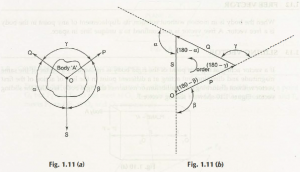According to the law of the triangle of forces, if there are three concurrent forces, which are coplanar, act on a rigid form of a body and keep it in the state of equilibrium, then the forces represent its direction and magnitude by the sides of a triangle. Here, the three concurrent sides are there with three different sides of a triangle and these sides are taken in an order.The explanation is important to understand the fact and here you can easily see that three forces act on a point O gets joined through the dotted line and with the help of the formula of a triangle, it can easily be evaluated.

In case of two forces acting on a point represented by two sided of a triangle, and then the third side can give you the resultant of those forces. In this way, the triangle rule expresses the resultant, but here the outcome can be seen for the two forces only.

Links of Previous Main Topic:-

Links of Next Mechanical Engineering Topics:-

### Submit Your Assignment### Customer Reviews

My Homework Help
Rated 5.0 out of 5 based on 510 customer reviews at